To understand  the equation of a plane motion of a rigid body, consider a rigid body moving in X-Y plane. So, here is the diagram to make the things convenient. The center of mass G has the acceleration ‘ a’. The angular velocity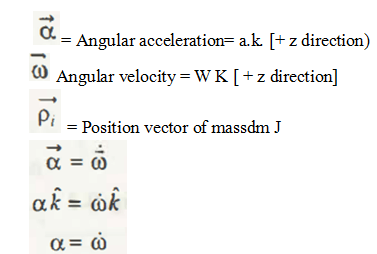The angular momentum of mass center G for the particle m can be represented as a scalar equation and, finally, it is clear through this equation that the angular acceleration is same as the rate of change of angular velocity with time.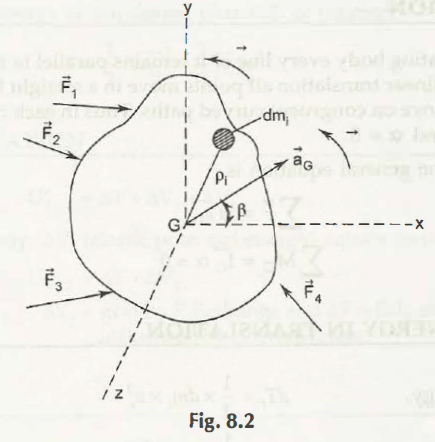Angular momentum can be expressed as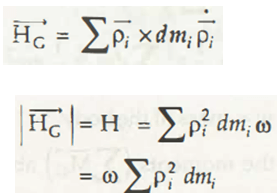So, the conclusion is HG=  wIG. It means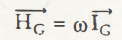Now, from all the equations, it is clear that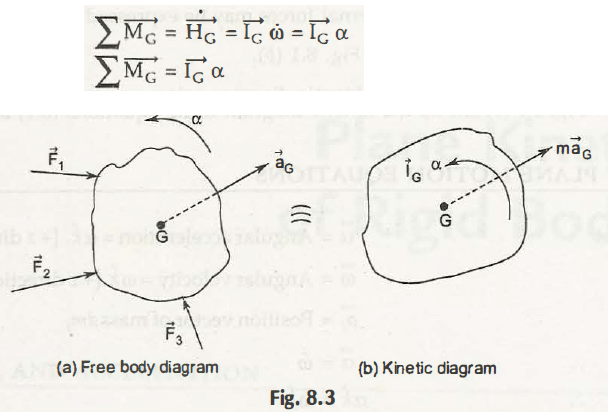The term I, is a constant and measure the inertia of rotation. IG is the mass moment of inertia about G or the mass center. It is also known as the moment of inertia of mass M through Z axis. Now, through the above explanation you can easily get that how to explain x-y Plane Motion Equations.

Links of Next Mechanical Engineering Topics:-### Customer Reviews

My Homework Help
Rated 5.0 out of 5 based on 510 customer reviews at